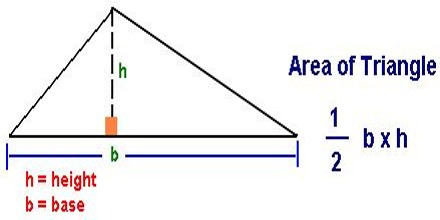Calculating the Area of a Triangle

The area of a triangle can be found by multiplying the base times the one-half the height.

To find the area of a triangle, multiply the base by the height, and then divide by 2. The division by 2 comes from the fact that a parallelogram can be divided into 2 triangles.

Since the area of a parallelogram is A = b x h, the area of a triangle must be one-half the area of a parallelogram. Thus, the formula for the area of a triangle is: A = ½ b * hIf a triangle has a base of length 6 inches and a height of 4 inches, its area is [½ (6 x 4)] = 12 square inches.

The base and height of a triangle must be perpendicular to each other. In each of the examples below, the base is a side of the triangle. However, depending on the triangle, the height may or may not be a side of the triangle.

Example: Find the area of an acute triangle with a base of 15 inches and a height of 4 inches.

Solution: A = ½ b * h

= ½ * (15 in) * (4 in)

= ½ (60 in2)

= 30 in2.